LR  >  Series Quiz II, Non Verbal Reasoning

# Series Quiz II, Non Verbal Reasoning

Test Description

## 25 Questions MCQ Test Non Verbal Reasoning | Series Quiz II, Non Verbal Reasoning

Series Quiz II, Non Verbal Reasoning for LR 2022 is part of Non Verbal Reasoning preparation. The Series Quiz II, Non Verbal Reasoning questions and answers have been prepared according to the LR exam syllabus.The Series Quiz II, Non Verbal Reasoning MCQs are made for LR 2022 Exam. Find important definitions, questions, notes, meanings, examples, exercises, MCQs and online tests for Series Quiz II, Non Verbal Reasoning below.
Solutions of Series Quiz II, Non Verbal Reasoning questions in English are available as part of our Non Verbal Reasoning for LR & Series Quiz II, Non Verbal Reasoning solutions in Hindi for Non Verbal Reasoning course. Download more important topics, notes, lectures and mock test series for LR Exam by signing up for free. Attempt Series Quiz II, Non Verbal Reasoning | 25 questions in 50 minutes | Mock test for LR preparation | Free important questions MCQ to study Non Verbal Reasoning for LR Exam | Download free PDF with solutions
 1 Crore+ students have signed up on EduRev. Have you?
Series Quiz II, Non Verbal Reasoning - Question 1

### Directions to Solve Each of the following questions consists of five figures marked A, B, C, D and E called the Problem Figures followed by five other figures marked 1, 2, 3, 4 and 5 called the Answer Figures. Select a figure from amongst the Answer Figures which will continue the same series as established by the five Problem Figures. Question -   Select a figure from amongst the Answer Figures which will continue the same series as established by the five Problem Figures. Problem Figures:                          Answer Figures: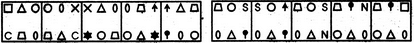(A)     (B)     (C)     (D)     (E)                (1)     (2)     (3)     (4)     (5)

Detailed Solution for Series Quiz II, Non Verbal Reasoning - Question 1

The elements move in the sequences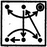and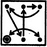alternately. Also, in each step, the symbol that reaches the encircled position, gets replaced by a new symbol.

Series Quiz II, Non Verbal Reasoning - Question 2

### Directions to Solve Each of the following questions consists of five figures marked A, B, C, D and E called the Problem Figures followed by five other figures marked 1, 2, 3, 4 and 5 called the Answer Figures. Select a figure from amongst the Answer Figures which will continue the same series as established by the five Problem Figures. Question - Select a figure from amongst the Answer Figures which will continue the same series as established by the five Problem Figures.Problem Figures:                          Answer Figures: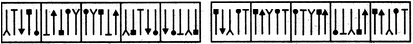(A)     (B)     (C)     (D)     (E)       (1)     (2)     (3)     (4)     (5)

Detailed Solution for Series Quiz II, Non Verbal Reasoning - Question 2 In one step, all the elements get vertically inverted and interchange positions in the sequence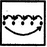. In the next step, the elements interchange positions in the sequence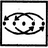and the third element gets vertically inverted.
Series Quiz II, Non Verbal Reasoning - Question 3

### Directions to Solve Each of the following questions consists of five figures marked A, B, C, D and E called the Problem Figures followed by five other figures marked 1, 2, 3, 4 and 5 called the Answer Figures. Select a figure from amongst the Answer Figures which will continue the same series as established by the five Problem Figures. Question - Select a figure from amongst the Answer Figures which will continue the same series as established by the five Problem Figures.Problem Figures:                          Answer Figures: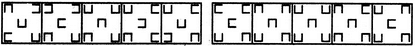(A)     (B)     (C)     (D)     (E)       (1)     (2)     (3)     (4)     (5)

Detailed Solution for Series Quiz II, Non Verbal Reasoning - Question 3 Three elements (encircled in-the figures below) rotate through 90oCW in each step. This rotation takes place in the following sequence :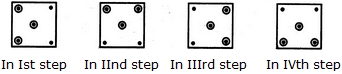This sequence is repeated to continue the series.
Series Quiz II, Non Verbal Reasoning - Question 4
Directions to Solve

Each of the following questions consists of five figures marked A, B, C, D and E called the Problem Figures followed by five other figures marked 1, 2, 3, 4 and 5 called the Answer Figures. Select a figure from amongst the Answer Figures which will continue the same series as established by the five Problem Figures.

Question -

Select a figure from amongst the Answer Figures which will continue the same series as established by the five Problem Figures.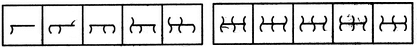(A)     (B)     (C)     (D)     (E)       (1)     (2)     (3)     (4)     (5)
Detailed Solution for Series Quiz II, Non Verbal Reasoning - Question 4 In each step, all the existing arcs get laterally inverted and a new arc is added which is oriented in a direction opposite to that of the last added arc. The arcs are added at various positions in the following sequences :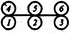Series Quiz II, Non Verbal Reasoning - Question 5
Directions to Solve

Each of the following questions consists of five figures marked A, B, C, D and E called the Problem Figures followed by five other figures marked 1, 2, 3, 4 and 5 called the Answer Figures. Select a figure from amongst the Answer Figures which will continue the same series as established by the five Problem Figures.

Question -

Select a figure from amongst the Answer Figures which will continue the same series as established by the five Problem Figures.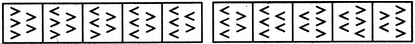(A)     (B)     (C)     (D)     (E)       (1)     (2)     (3)     (4)     (5)
Detailed Solution for Series Quiz II, Non Verbal Reasoning - Question 5 In each step, one of the elements-gets laterally inverted.
Series Quiz II, Non Verbal Reasoning - Question 6

Directions to Solve

Each of the following questions consists of five figures marked A, B, C, D and E called the Problem Figures followed by five other figures marked 1, 2, 3, 4 and 5 called the Answer Figures. Select a figure from amongst the Answer Figures which will continue the same series as established by the five Problem Figures.

Question -

Select a figure from amongst the Answer Figures which will continue the same series as established by the five Problem Figures.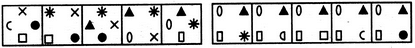(A)     (B)     (C)     (D)     (E)       (1)     (2)     (3)     (4)     (5)

Series Quiz II, Non Verbal Reasoning - Question 7
Directions to Solve

Each of the following questions consists of five figures marked A, B, C, D and E called the Problem Figures followed by five other figures marked 1, 2, 3, 4 and 5 called the Answer Figures. Select a figure from amongst the Answer Figures which will continue the same series as established by the five Problem Figures.

Question -

Select a figure from amongst the Answer Figures which will continue the same series as established by the five Problem Figures.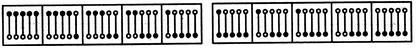(A)     (B)     (C)     (D)     (E)       (1)     (2)     (3)     (4)     (5)
Detailed Solution for Series Quiz II, Non Verbal Reasoning - Question 7 One of the pins gets inverted in each step. The pins get inverted sequentially from right to left.
Series Quiz II, Non Verbal Reasoning - Question 8
Directions to Solve

Each of the following questions consists of five figures marked A, B, C, D and E called the Problem Figures followed by five other figures marked 1, 2, 3, 4 and 5 called the Answer Figures. Select a figure from amongst the Answer Figures which will continue the same series as established by the five Problem Figures.

Question -

Select a figure from amongst the Answer Figures which will continue the same series as established by the five Problem Figures.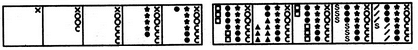(A)     (B)     (C)     (D)     (E)       (1)     (2)     (3)     (4)     (5)
Detailed Solution for Series Quiz II, Non Verbal Reasoning - Question 8 The number of symbols added sequentially is 3, 2, 5, 2, 7, 2, .... These symbols are added to form a sequence of 1, 2, 3, 4, 5, 6 identical symbols.
Series Quiz II, Non Verbal Reasoning - Question 9
Directions to Solve

Each of the following questions consists of five figures marked A, B, C, D and E called the Problem Figures followed by five other figures marked 1, 2, 3, 4 and 5 called the Answer Figures. Select a figure from amongst the Answer Figures which will continue the same series as established by the five Problem Figures.

Question -

Select a figure from amongst the Answer Figures which will continue the same series as established by the five Problem Figures.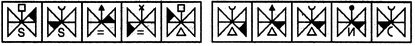(A)     (B)     (C)     (D)     (E)       (1)     (2)     (3)     (4)     (5)
Detailed Solution for Series Quiz II, Non Verbal Reasoning - Question 9 The shading moves in the sequence as shown in the figure: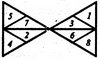The similar upper element appears in every fourth step. The lower element is replaced by a new element in every second step.
Series Quiz II, Non Verbal Reasoning - Question 10
Directions to Solve

Each of the following questions consists of five figures marked A, B, C, D and E called the Problem Figures followed by five other figures marked 1, 2, 3, 4 and 5 called the Answer Figures. Select a figure from amongst the Answer Figures which will continue the same series as established by the five Problem Figures.

Question -

Select a figure from amongst the Answer Figures which will continue the same series as established by the five Problem Figures.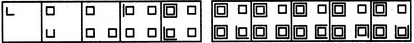(A)     (B)     (C)     (D)     (E)       (1)     (2)     (3)     (4)     (5)
Detailed Solution for Series Quiz II, Non Verbal Reasoning - Question 10 Five line segments are added in each step to complete the squares in an ACW direction.
Series Quiz II, Non Verbal Reasoning - Question 11
Directions to Solve

Each of the following questions consists of five figures marked A, B, C, D and E called the Problem Figures followed by five other figures marked 1, 2, 3, 4 and 5 called the Answer Figures. Select a figure from amongst the Answer Figures which will continue the same series as established by the five Problem Figures.

Question -

Select a figure from amongst the Answer Figures which will continue the same series as established by the five Problem Figures.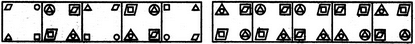(A)     (B)     (C)     (D)     (E)       (1)     (2)     (3)     (4)     (5)
Detailed Solution for Series Quiz II, Non Verbal Reasoning - Question 11 Similar figure appears alternately and each time a figure reappears, all the elements move one step CW.
Series Quiz II, Non Verbal Reasoning - Question 12
Directions to Solve

Each of the following questions consists of five figures marked A, B, C, D and E called the Problem Figures followed by five other figures marked 1, 2, 3, 4 and 5 called the Answer Figures. Select a figure from amongst the Answer Figures which will continue the same series as established by the five Problem Figures.

Question -

Select a figure from amongst the Answer Figures which will continue the same series as established by the five Problem Figures.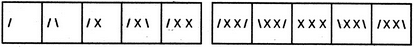(A)     (B)     (C)     (D)     (E)       (1)     (2)     (3)     (4)     (5)
Detailed Solution for Series Quiz II, Non Verbal Reasoning - Question 12 One extra line is added in each step in a set order.
Series Quiz II, Non Verbal Reasoning - Question 13
Directions to Solve

Each of the following questions consists of five figures marked A, B, C, D and E called the Problem Figures followed by five other figures marked 1, 2, 3, 4 and 5 called the Answer Figures. Select a figure from amongst the Answer Figures which will continue the same series as established by the five Problem Figures.

Question -

Select a figure from amongst the Answer Figures which will continue the same series as established by the five Problem Figures.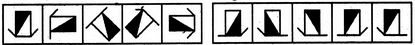(A)     (B)     (C)     (D)     (E)       (1)     (2)     (3)     (4)     (5)
Detailed Solution for Series Quiz II, Non Verbal Reasoning - Question 13 Similar figure reappears in every fourth step and each time a figure reappears, it rotates through 90oACW.
Series Quiz II, Non Verbal Reasoning - Question 14
Directions to Solve

Each of the following questions consists of five figures marked A, B, C, D and E called the Problem Figures followed by five other figures marked 1, 2, 3, 4 and 5 called the Answer Figures. Select a figure from amongst the Answer Figures which will continue the same series as established by the five Problem Figures.

Question -

Select a figure from amongst the Answer Figures which will continue the same series as established by the five Problem Figures.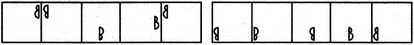(A)     (B)     (C)     (D)     (E)       (1)     (2)     (3)     (4)     (5)
Detailed Solution for Series Quiz II, Non Verbal Reasoning - Question 14 The symbol gets vertically inverted and laterally inverted alternately. It also moves in ACW direction through distances equal to two half-sides (of square boundary) and three half-sides alternately.
Series Quiz II, Non Verbal Reasoning - Question 15
Directions to Solve

Each of the following questions consists of five figures marked A, B, C, D and E called the Problem Figures followed by five other figures marked 1, 2, 3, 4 and 5 called the Answer Figures. Select a figure from amongst the Answer Figures which will continue the same series as established by the five Problem Figures.

Question -

Select a figure from amongst the Answer Figures which will continue the same series as established by the five Problem Figures.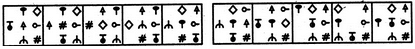(A)     (B)     (C)     (D)     (E)       (1)     (2)     (3)     (4)     (5)
Detailed Solution for Series Quiz II, Non Verbal Reasoning - Question 15 In one step, the elements move in the sequence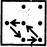and in the next step, the elements move in the sequence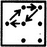The two steps are repeated alternately.
Series Quiz II, Non Verbal Reasoning - Question 16
Directions to Solve

Each of the following questions consists of five figures marked A, B, C, D and E called the Problem Figures followed by five other figures marked 1, 2, 3, 4 and 5 called the Answer Figures. Select a figure from amongst the Answer Figures which will continue the same series as established by the five Problem Figures.

Question -

Select a figure from amongst the Answer Figures which will continue the same series as established by the five Problem Figures.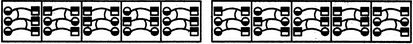(A)     (B)     (C)     (D)     (E)       (1)     (2)     (3)     (4)     (5)
Detailed Solution for Series Quiz II, Non Verbal Reasoning - Question 16 The elements interchange positions in the orders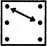and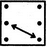alternately.
Series Quiz II, Non Verbal Reasoning - Question 17
Directions to Solve

Each of the following questions consists of five figures marked A, B, C, D and E called the Problem Figures followed by five other figures marked 1, 2, 3, 4 and 5 called the Answer Figures. Select a figure from amongst the Answer Figures which will continue the same series as established by the five Problem Figures.

Question -

Select a figure from amongst the Answer Figures which will continue the same series as established by the five Problem Figures.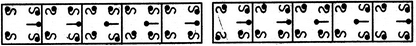(A)     (B)     (C)     (D)     (E)       (1)     (2)     (3)     (4)     (5)
Detailed Solution for Series Quiz II, Non Verbal Reasoning - Question 17 The upper-left element gets laterally inverted in first, third, fifth. .... steps; the upper-right element gets rotated through 180o is first, fourth, seventh,.... steps; the lower-left element gets laterally inverted in second, fourth, sixth, ... steps; the lower-right element gets rotated through 180o in third, sixth,... steps and the pin at the middle-right position gets laterally inverted in every second step.
Series Quiz II, Non Verbal Reasoning - Question 18
Directions to Solve

Each of the following questions consists of five figures marked A, B, C, D and E called the Problem Figures followed by five other figures marked 1, 2, 3, 4 and 5 called the Answer Figures. Select a figure from amongst the Answer Figures which will continue the same series as established by the five Problem Figures.

Question -

Select a figure from amongst the Answer Figures which will continue the same series as established by the five Problem Figures.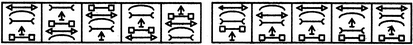(A)     (B)     (C)     (D)     (E)       (1)     (2)     (3)     (4)     (5)
Detailed Solution for Series Quiz II, Non Verbal Reasoning - Question 18 In each step, the elements move in the order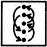Series Quiz II, Non Verbal Reasoning - Question 19
Directions to Solve

Each of the following questions consists of five figures marked A, B, C, D and E called the Problem Figures followed by five other figures marked 1, 2, 3, 4 and 5 called the Answer Figures. Select a figure from amongst the Answer Figures which will continue the same series as established by the five Problem Figures.

Question -

Select a figure from amongst the Answer Figures which will continue the same series as established by the five Problem Figures.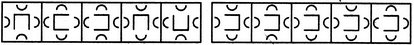(A)     (B)     (C)     (D)     (E)       (1)     (2)     (3)     (4)     (5)
Detailed Solution for Series Quiz II, Non Verbal Reasoning - Question 19 Three and two arcs are inverted alternately. The central element rotates 90oACW and 180o alternately.
Series Quiz II, Non Verbal Reasoning - Question 20
Directions to Solve

Each of the following questions consists of five figures marked A, B, C, D and E called the Problem Figures followed by five other figures marked 1, 2, 3, 4 and 5 called the Answer Figures. Select a figure from amongst the Answer Figures which will continue the same series as established by the five Problem Figures.

Question -

Select a figure from amongst the Answer Figures which will continue the same series as established by the five Problem Figures.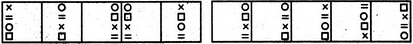(A)     (B)     (C)     (D)     (E)       (1)     (2)     (3)     (4)     (5)
Detailed Solution for Series Quiz II, Non Verbal Reasoning - Question 20 All the elements together move one space to the right in each step and once they reach the rightmost position, then in the next step, they move to the leftmost position. Also, in the first step, the first (uppermost) and the third elements interchange positions; in the second step, the second and the fourth elements interchange positions and in the third step, none of the elements interchange positions. These three steps are repeated to continue the series.
Series Quiz II, Non Verbal Reasoning - Question 21
Directions to Solve

Each of the following questions consists of five figures marked A, B, C, D and E called the Problem Figures followed by five other figures marked 1, 2, 3, 4 and 5 called the Answer Figures. Select a figure from amongst the Answer Figures which will continue the same series as established by the five Problem Figures.

Question -

Select a figure from amongst the Answer Figures which will continue the same series as established by the five Problem Figures.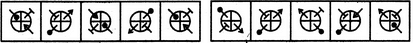(A)     (B)     (C)     (D)     (E)       (1)     (2)     (3)     (4)     (5)
Detailed Solution for Series Quiz II, Non Verbal Reasoning - Question 21 All the elements move one space ACW (each space is equal to a quadrant of the circle) and get inverted in each step.
Series Quiz II, Non Verbal Reasoning - Question 22
Directions to Solve

Each of the following questions consists of five figures marked A, B, C, D and E called the Problem Figures followed by five other figures marked 1, 2, 3, 4 and 5 called the Answer Figures. Select a figure from amongst the Answer Figures which will continue the same series as established by the five Problem Figures.

Question -

Select a figure from amongst the Answer Figures which will continue the same series as established by the five Problem Figures.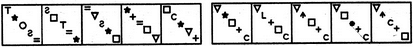(A)     (B)     (C)     (D)     (E)       (1)     (2)     (3)     (4)     (5)
Detailed Solution for Series Quiz II, Non Verbal Reasoning - Question 22 In each step, the symbols move in the sequence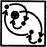and the symbol that reaches the encircled position gets replaced by a new one.
Series Quiz II, Non Verbal Reasoning - Question 23
Directions to Solve

Each of the following questions consists of five figures marked A, B, C, D and E called the Problem Figures followed by five other figures marked 1, 2, 3, 4 and 5 called the Answer Figures. Select a figure from amongst the Answer Figures which will continue the same series as established by the five Problem Figures.

Question -

Select a figure from amongst the Answer Figures which will continue the same series as established by the five Problem Figures.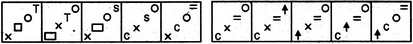(A)     (B)     (C)     (D)     (E)       (1)     (2)     (3)     (4)     (5)
Detailed Solution for Series Quiz II, Non Verbal Reasoning - Question 23 The elements move in the sequences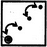and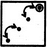alternately. Also, in each step, the element that reaches the encircled position, gets replaced by a new element.
Series Quiz II, Non Verbal Reasoning - Question 24
Directions to Solve

Each of the following questions consists of five figures marked A, B, C, D and E called the Problem Figures followed by five other figures marked 1, 2, 3, 4 and 5 called the Answer Figures. Select a figure from amongst the Answer Figures which will continue the same series as established by the five Problem Figures.

Question -

Select a figure from amongst the Answer Figures which will continue the same series as established by the five Problem Figures.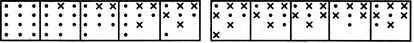(A)     (B)     (C)     (D)     (E)       (1)     (2)     (3)     (4)     (5)
Detailed Solution for Series Quiz II, Non Verbal Reasoning - Question 24 In each step, one dot is lost while another dot is replaced by a cross.
Series Quiz II, Non Verbal Reasoning - Question 25
Directions to Solve

Each of the following questions consists of five figures marked A, B, C, D and E called the Problem Figures followed by five other figures marked 1, 2, 3, 4 and 5 called the Answer Figures. Select a figure from amongst the Answer Figures which will continue the same series as established by the five Problem Figures.

Question -

Select a figure from amongst the Answer Figures which will continue the same series as established by the five Problem Figures.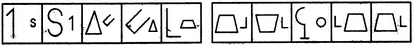(A)     (B)     (C)     (D)     (E)       (1)     (2)     (3)     (4)     (5)
Detailed Solution for Series Quiz II, Non Verbal Reasoning - Question 25 In one step, the two elements interchange positions and the smaller element gets enlarged while the larger element gets reduced in size. In the next step, the smaller element is replaced by a new small element and the larger element is replaced by a new large element.

## Non Verbal Reasoning

40 tests
 Use Code STAYHOME200 and get INR 200 additional OFF Use Coupon Code
Information about Series Quiz II, Non Verbal Reasoning Page
In this test you can find the Exam questions for Series Quiz II, Non Verbal Reasoning solved & explained in the simplest way possible. Besides giving Questions and answers for Series Quiz II, Non Verbal Reasoning, EduRev gives you an ample number of Online tests for practice

40 tests